# Triangle ABC

Construct a triangle ABC is is given c = 60mm hc = 40 mm and b = 48 mm
analysis
procedure steps
construction

a =  52.154 mm

### Step-by-step explanation:

Try calculation via our triangle calculator.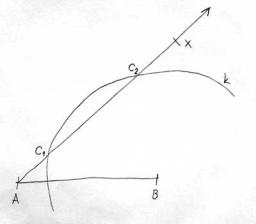Did you find an error or inaccuracy? Feel free to write us. Thank you!Tips to related online calculators

#### You need to know the following knowledge to solve this word math problem:

We encourage you to watch this tutorial video on this math problem:

## Related math problems and questions:

• Triangle SSAConstruct a triangle ABC if |AB| = 5cm va = 3cm, CAB = 50 °. It is to create the analysis and construction steps.
• Construction of trapezoidConstruct a trapezoid if b = 4cm, c = 7cm, d = 4,5cm, v = 3 cm (Procedure, discussion, sketch, analysis, construction)
• Ruler and compassUse a ruler and compass to construct a triangle ABC with AB 5cm BAC 60° and ACB 45°.
• Construction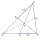Construction the triangle ABC, if you know: the size of the side AC is 6 cm, the size of the angle ACB is 60° and the distance of the center of gravity T from the vertex A is 4 cm. (Sketch, analysis, notation of construction, construction)
• Draw triangle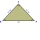Construct an isosceles triangle ABC, if AB = 7cm, the size of the angle ABC is 47°, arms | AC | = | BC |. Measure the size of the BC side in mm.
• ConstructConstruct a triangle ABC inscribed circle has a radius r = 2 cm, the angle alpha = 50 degrees = 8 cm. Make a sketch, analysis, construction and description.
• Construct 1Construct a triangle ABC, a = 7 cm, b = 9 cm with right angle at C, construct the axis of all three sides. Measure the length of side c (and write).
• Trapezoid - constructionConstruct a trapezoid KLMN, where: k = 9 cm, l = 4 cm, m = 5 cm and angle α = 45°
• Complete constructionConstruct triangle ABC if hypotenuse c = 7 cm and angle ABC = 30 degrees. / Use Thales' theorem - circle /. Measure and write down the length of legs.
• Sides od triangleSides of the triangle ABC has length 4 cm, 5 cm and 7 cm. Construct triangle A'B'C' that are similar to triangle ABC which has a circumference of 12 cm.
• Tangents constructThe circle k is given k (S; 2.5 cm) and an outer line p. Construct a tangent t of the circle that has with a line p angle 60°. How many solutions have the task?
• Draw a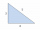Draw a triangle ABC, if you know: alpha = 60° side b = 4 cm side a = 10 cm
• Resultant forceCalculate mathematically and graphically the resultant of three forces with a common center if: F1 = 50 kN α1 = 30° F2 = 40 kN α2 = 45° F3 = 40 kN α3 = 25°
• Isosceles - isoscelesIt is given a triangle ABC with sides /AB/ = 3 cm /BC/ = 10 cm, and the angle ABC = 120°. Draw all points X such that true that BCX triangle is an isosceles and triangle ABX is isosceles with the base AB.
• Construct rhombusConstruct rhombus ABCD if given diagonal length | AC | = 8cm, inscribed circle radius r = 1.5cm
• Two heights and a side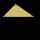Construct triangle ABC when the given side is c = 7 cm, height to side a va = 5 cm and height to side b: vb = 4 cm.
• Reverse Pythagorean theoremGiven are lengths of the sides of the triangles. Decide which one is rectangular: Δ ABC: 77 dm, 85 dm, 36 dm ... Δ DEF: 55 dm, 82 dm, 61 dm ... Δ GHI: 24 mm, 25 mm, 7 mm ... Δ JKL: 32 dm, 51 dm, 82 dm ... Δ MNO: 51 dm, 45 dm,